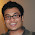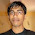### Pile Puzzle

Problem:
You have 55 matches arranged in some number of piles of different sizes. You now do the following operation: pick one match from each pile, and form a new pile. You repeat this ad infinitum. What is the steady state? Is it unique?

Update (15th Feb 2012):
Clarification:
Assume steady state exists and try to solve the problem.
A much difficult version: Prove that steady state always exists.

Solution:

Solution posted by Avradeep Bhowmik (aletterdoesnotblush), Sumanth Puram (IITM Alumnus, Engineer at Netapp), Mayur Agrawal (Agmay, IIT KGP Alumnus, PhD Student at Purdue University) in comments!

The difficult version posed by Nishant Totla (CSE IITB Senior Undergraduate) and solved by Gaurav Sinha (chera, IITK 1996 Graduate, Indian Revenue Service). Thanks a ton!

1.TO reach a steady state, the sizes of piles should be cyclic.
Sigma(10) = 55

Hence the steady state will be 1, 2, 3, .., 9, 10

The new pile that forms after every step has size 10 and the sizes of all existing piles reduce by 1 and become 1, 2, 3, .. 9.

2.Sizes of all piles should be cyclic.

Sigma (10) = 55.
Hence the steady state will be 1, 2, 3, .. , 9, 10.

After every step, each existing pile size will reduce by 1 and the new pile size will be equal to 10.

3.Say there were N piles in the steady state. We can say the following-

1. There must be exactly one pile containing only 1 match, since one pile must be lost as one extra pile is being generated.

2. At steady state, every pile remaining/constructed after performing the operation must correspond exactly to those before the operation.

3. The new pile contains N matches. Thus there must exist a pile in the original setup (before the operation) containing N piles. Hence, after the operation it contains (N-1) matches, thus the original setup must have an (N-1)-match pile which reduced to (N-2)-match pile, and so on. Every pile can be enumerated this way. [Otherwise, for any other pile of, say, k matches, we get a similar decreasing sequence terminating in 1. A construction of a new pile from M such sequences will lead to M piles disappearing, but only 1 will be created. Hence, M=1]

Therefore we have, from the unique sequence, N + (N-1) + ... + 1 = 55 which gives the value of N as 10.

And by the above reasoning, this is unique.

4.At steady state, we would expect the number of piles to remain constant. However after each iteration, we create a new pile. Therefore, exactly one of the piles should have only 1 match stick before we start the next iteration. Arguing similarly, we can construct the optimal set to be (1,2,....10). The uniqueness follows from the recursive argument given above.

5.It is easy to show that a steady state exists, and is unique too. But can we show that the steady state will always be reached?

6.One question about the problem though: Are we guaranteed to reach the steady state, no matter what initial state we start with? Or is it possible that we may get entangled in a loop, in which none of the state corresponds to the steady state?

What everyone has shown here is that (1,2,...10) is the unique absorbing state. However that does not guarantee its reachability from any starting point. Am I missing something here?

7.infiniti and Agmay: Reachability follows from

i) The finiteness of the number of states.
ii) The fact that the next state is completely determined, given the previous state.

8.I have some interesting observations, mainly from computer runs.

The stable state/cycle lengths for sample heaps with sizes 1,2,3,4,5,6 ... were:
1,2,1,3,3,1,4,4,4,1,5,5,5,5,1 ...
nice order, not sure if this is true for every heap made from partitioning a 'n' number of matches.

Also in most of the heaps of 'n' matches, stable state/cycle were same. Again not sure if this is true.

Apologies if I am misleading anyone in a wrong path.

9.Can someone prove that the steady state is reachable from any initial state?

I don't think that Pseudonymous' proof is correct (or is it?).

10.I too think its not correct!

11.My apologies -- I didn't fully read the comments and thought it had been proved that {1, 2, ..., 10} was the only *dynamic* steady state, in which case reachability follows trivially.

12.proof that {1,2,3,....,10} is reachable from any starting configuration. proof can be adapted to show that if we start from N matches, where N= 1+2+...+k +r, where 0< r< k+1, then we get into a cycle where there are k or k+1 piles in each state,each pile is of size at most k+1, and the length of cycle is divisor of k+1.

suppose to the contrary that (1,2,3,...,10) is not reachable. As number of states is finite, ultimately we must reach a cycle.

suppose cycle is X_1->X_2->X_3....->X_L-> X_1... where L (>1) is length of cycle and Xi s are the various states. Let b(i) denote number of piles in state X_i. if i<=0 or i>L, define b(i) as b(j) where j=i modulo L, and 0< j < = L.

observe that each pile element in X_i is derived from a b(j) where j< i. thus in state X_i pile sizes will be b(i-1), b(i-2)-1,b(i-3)-2,..., where we choose the numbers that are positive and we discard numbers <= 0.

suppose k=minimum {b(i), i = 1 to L}.

k+r= maximum (b(i), i = 1 to L}.
now, r>0, for if r = 0, it can be easily shown that all states contain 10 piles of sizes {1,2,3,…,10}.

Claim1: if b(i)=k , then b(i-(k+1))=k.
Claim2: if b(j)=k+r, then b(j-(k+r))=k+r.
Proof of above claims is not detailed here but easily follows from fact that k and k+r are minimum and maximum sizes, and observation that

in state X_i pile sizes will be b(i-1), b(i-2)-1,b(i-3)-2,..., where we choose the numbers that are positive and we discard numbers <= 0.

Assume wlog that b(L)=k+r, and b(z)=k. where 1<= z< L.
Denote U= L-a(k+r), and V= z-a(k+1), where a is arbitrary whole number.

Claims above imply that b(U)= k+r, and b(V)=k .

Now, note that since the number of piles can increase by at most one in each step, Distance of directed segment/arc starting at V and ending at U >= r. this also implies L > r.

U-V = [ L-a(k+r)]-[ z-a(k+1)].
= L-z-a(r-1).
Now claim is that r=1, because if r is not equal to 1. , then divide L-z by r-1 to obtain L-z=q(r-1) +R, where 0<=R< r-1. Also, Since a was arbitrary, choose a=q,
We get U-V = q(r-1)+R-q(r-1)= R.

As R < r-1< L, so distance from V to U =R.

This yields contradiction i.e. r-1> R >=r.

So, in the cycle , at each state there are either k or k+1 piles.Further , b(i)=b(i-k-1) for all i, hence length of cycle L <= k+1 (in fact L is constrained to be a divisor of k+1).

Consider an arbitrary state having k piles.

Total number of matches in piles will be greater than k+(k-1)+(k-2)+….1= k(k+1)/2. (strictly greater than because,at least one state in the cycle will be of size k+1)

Further, total number of matches will be at most (k+1) +(k)+(k-1)+…+2 = k(k+1)/2+k.

We obtain inequality, k(k+1)/2 <55<= k(k+1)/2+k. this yields k<10<=k, a contradiction.

13.@chera.. Thanks a ton. This is amazing.